### Artificial Neural Networks

Dogs and cats are both furry animals with four legs and many other shared traits. Why, then, is it easy to distinguish between them?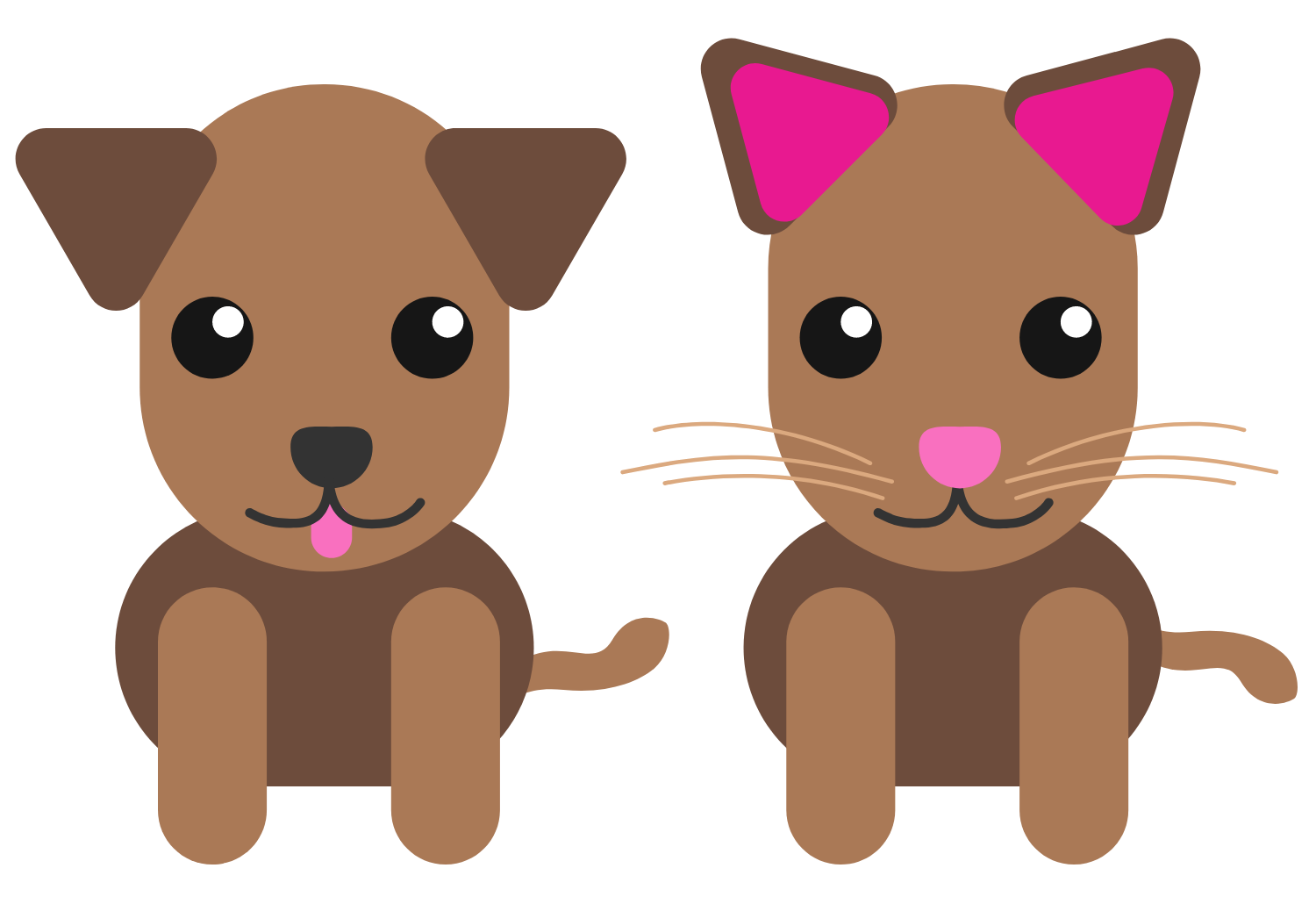As young people, we’re told which animals we observe are dogs and which are cats. Fairly quickly, we stop needing new examples. Our learning is powerful enough to classify a new animal as a dog or a cat, even when it doesn’t look particularly similar to one we’ve seen before. It turns out that computers can learn similarly.

# Learning Problems for Neural Networks

A supervised learning algorithm attempts to model a function to relate inputs to outputs. It uses known examples to learn this relationship.

When building a supervised learning model to distinguish whether an image is of a dog or a cat, what should the inputs for the examples be?

# Learning Problems for Neural Networks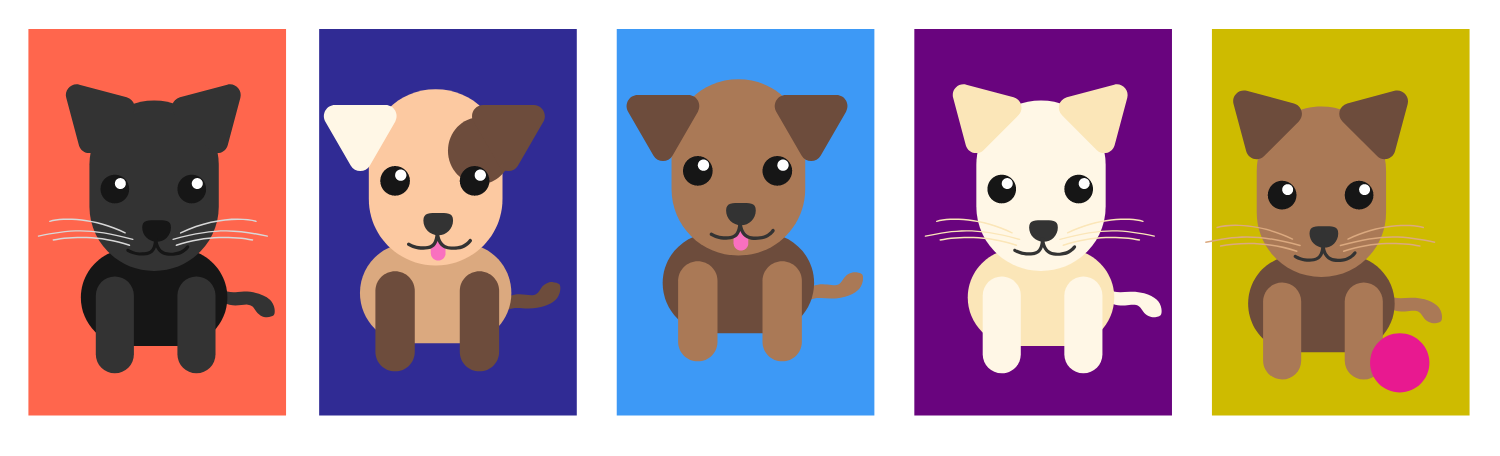Suppose you have access to 100,000 images of dogs and cats that you can use to build a supervised learning model that distinguishes between dogs and cats.

After using images as examples to train (or teach) the model, you’ll want to use images to test the model, that is, to determine if the model is actually successful at identifying if the image is of a dog or a cat.

What would be a reasonable way to select your images for training and testing?

# Learning Problems for Neural Networks

A good supervised learning model predicts the outputs of unobserved inputs using knowledge of the outputs of observed inputs. The ability to make successful predictions on unobserved inputs from observed data is called generalization.

For any observed data, there are an infinite number of functions that pass through all input-output pairs. The “best” function is not necessarily one which fits all of the observed data but, instead, it is one that generalizes well.

# Learning Problems for Neural Networks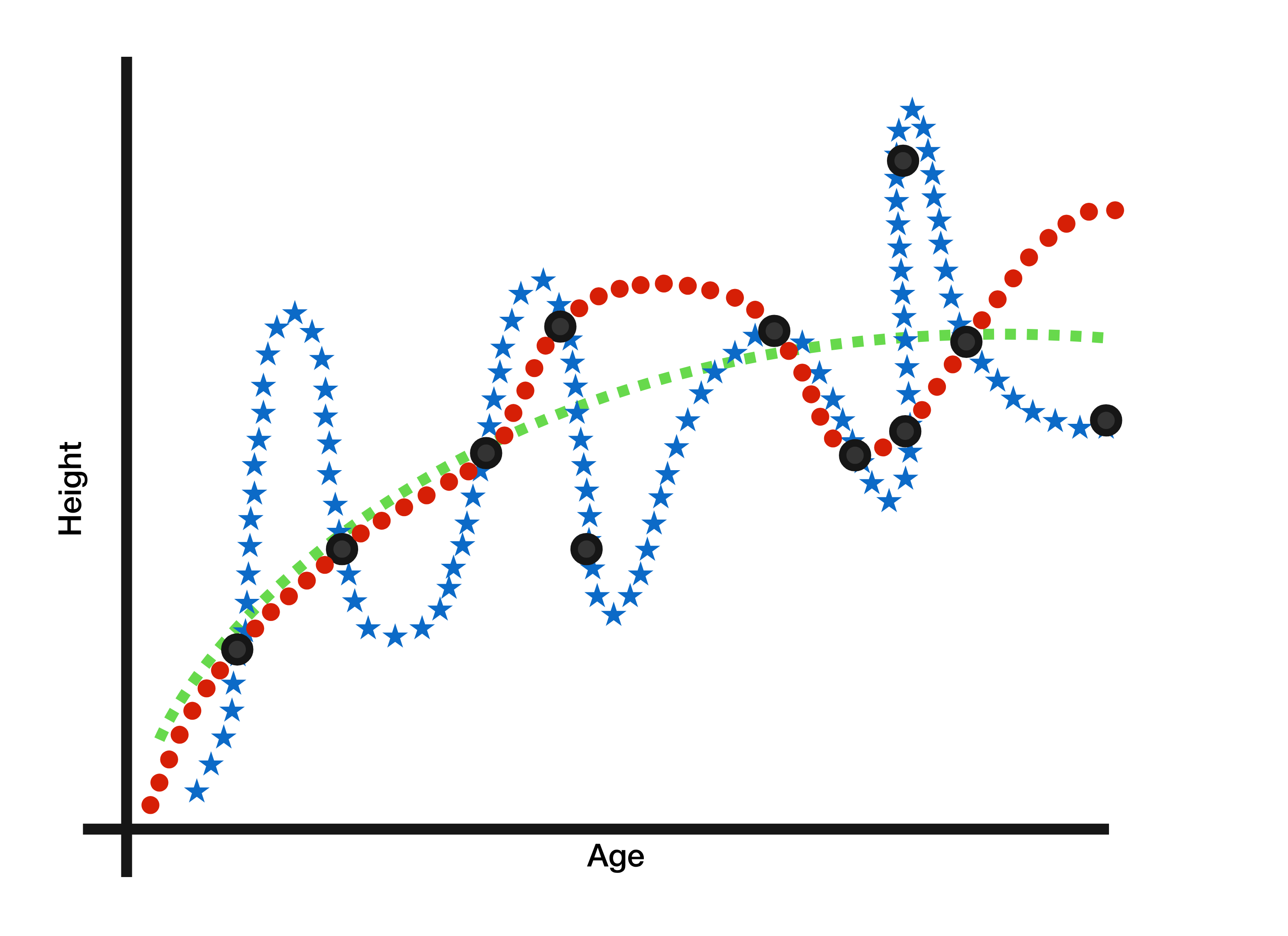You are training a height-prediction model using observed inputs of children's ages and outputs of their heights, shown as points in the graph above. Which of the three functions drawn is likely to be the best model?

# Learning Problems for Neural Networks

In the task of distinguishing between dogs and cats, we wanted to classify an image into discrete categories with no numerical relationship; i.e. we cannot say $\text {dog}$ is $2$ times $\text {cat}.$ This type of problem is called a classification problem.

On the other hand, in the previous question, we found a function to relate an input to a numerical output (height). These outputs have a clear numerical relationship; e.g. the output of $6$ feet is twice the output of $3$ feet. This type of problem is known as a regression problem.

Artificial neural networks (ANNs) are flexible enough to be used in both classification and regression problems.

# Learning Problems for Neural Networks

Some number of the following three learning problems are regression problems. Exactly how many count as regression problems?

• Identifying which Zip Code digits have been written on an envelope.
• Predicting the total number of points scored by two teams in a basketball game given the average age of the players.
• Determining the adult height of a child given his/her age and current height.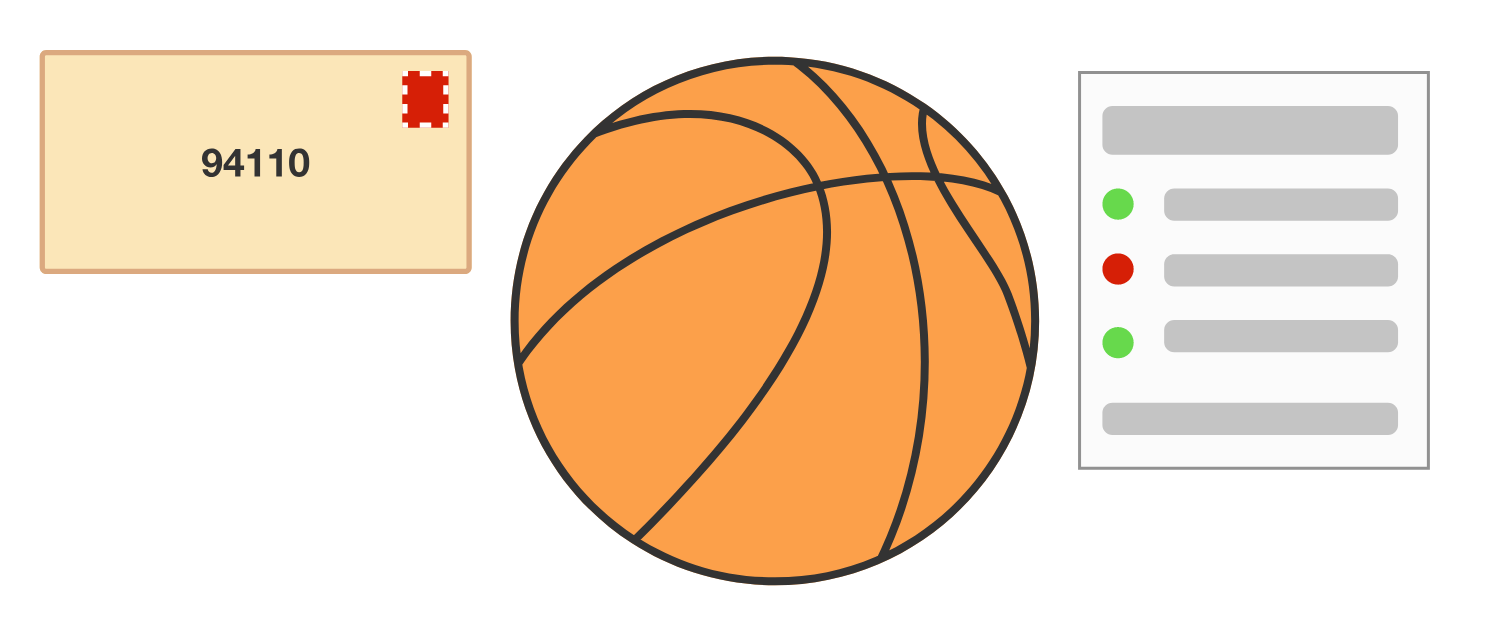# Learning Problems for Neural Networks

This quiz has focused on supervised learning problems, as many of the basic applications of ANNs involve supervised learning. However, there is another type of learning: unsupervised learning.

As the name might imply, unsupervised learning attempts to determine relationships between inputs without using any example outputs (such as “dog” or “cat” in our earlier example). Which of the following problems would be a good fit for supervised learning but a bad fit for unsupervised learning?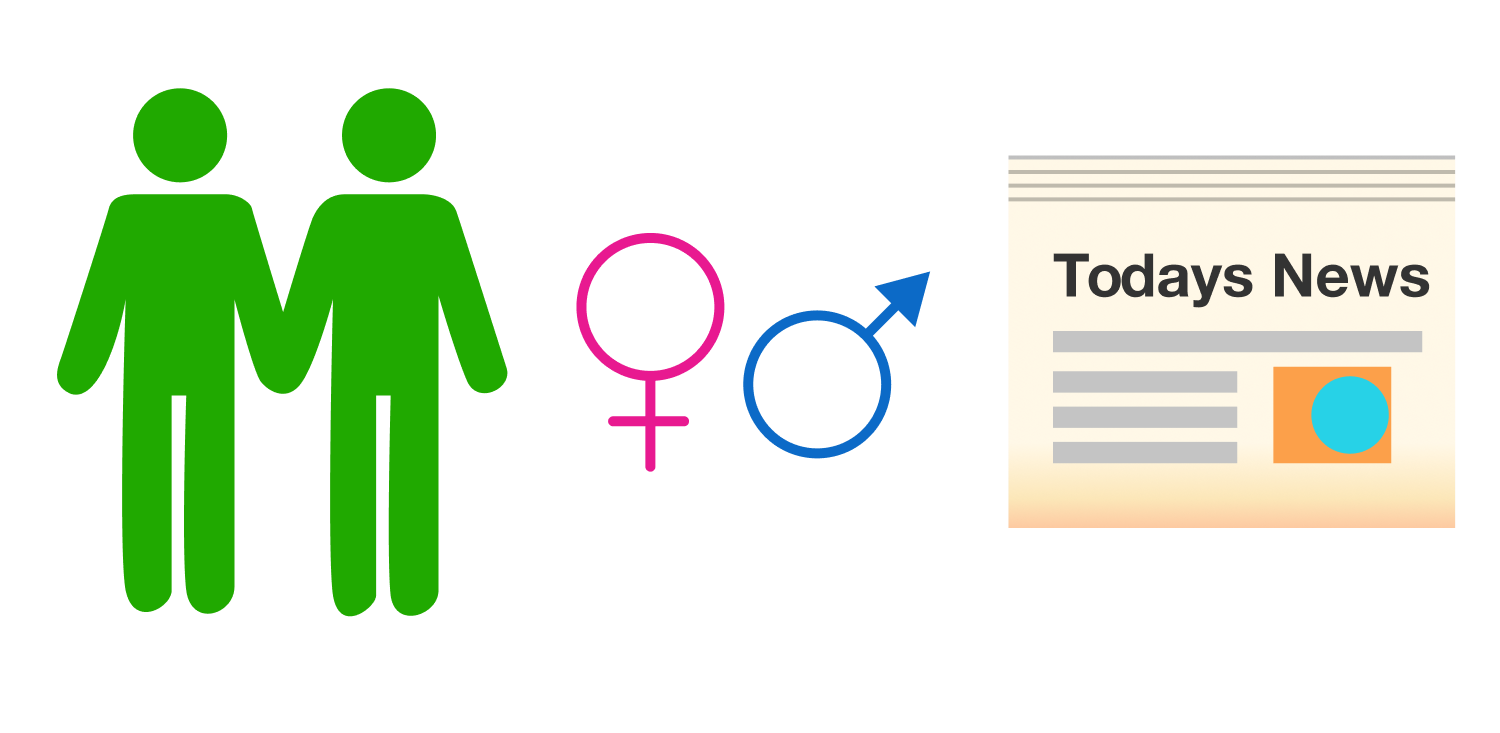# Learning Problems for Neural Networks

We now have a big-picture sense of what learning problems are all about. There are two main types of learning: unsupervised and supervised, and problems within supervised learning can be categorized as classification or regression problems. This course illustrates how to construct ANNs, a computational model that performs well in a wide variety of learning problems such as the ones discussed in this quiz.

In the next quiz, we’ll explore the basics of how the human brain works and how these ideas inspire the mechanisms within ANNs.

# Learning Problems for Neural Networks

×